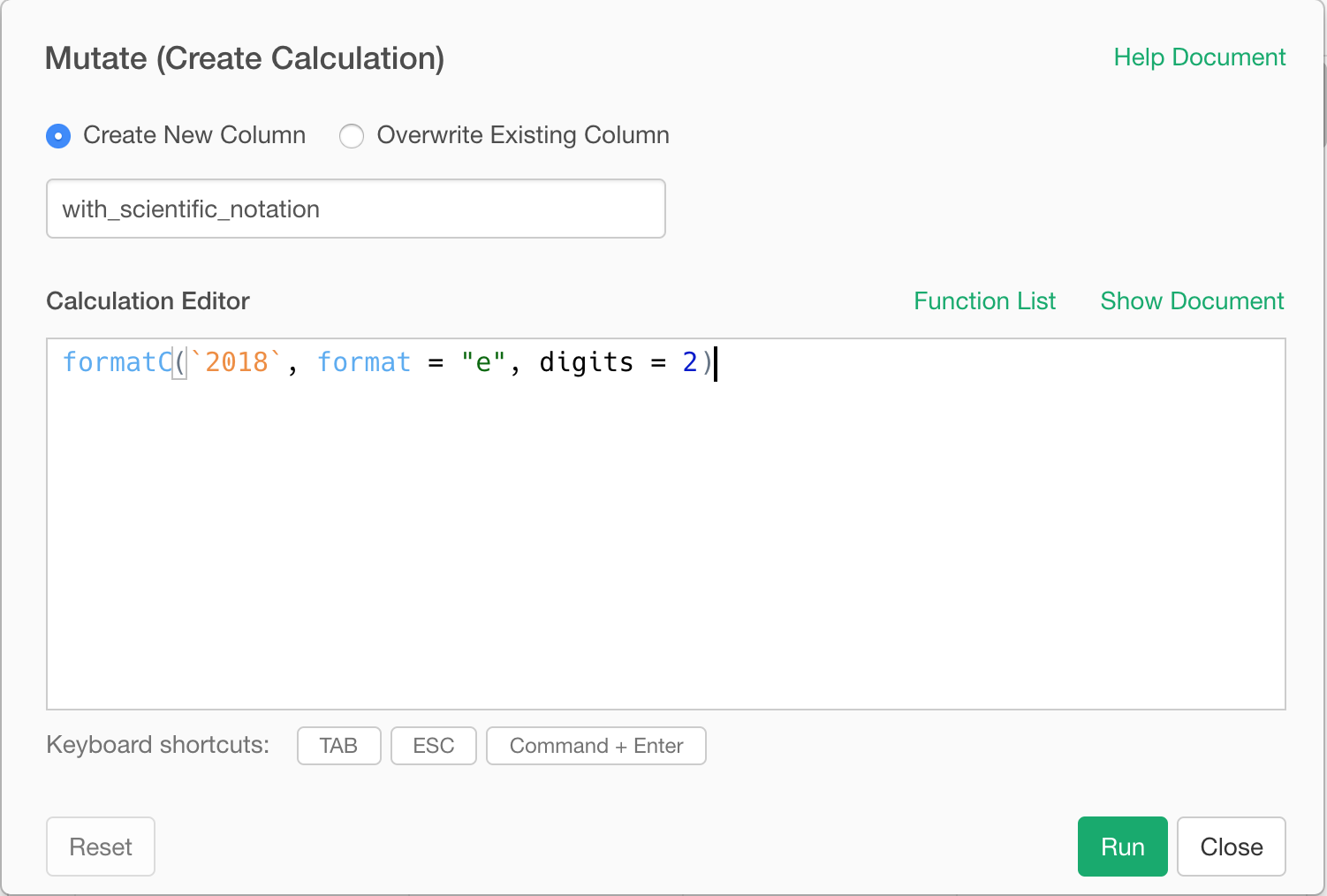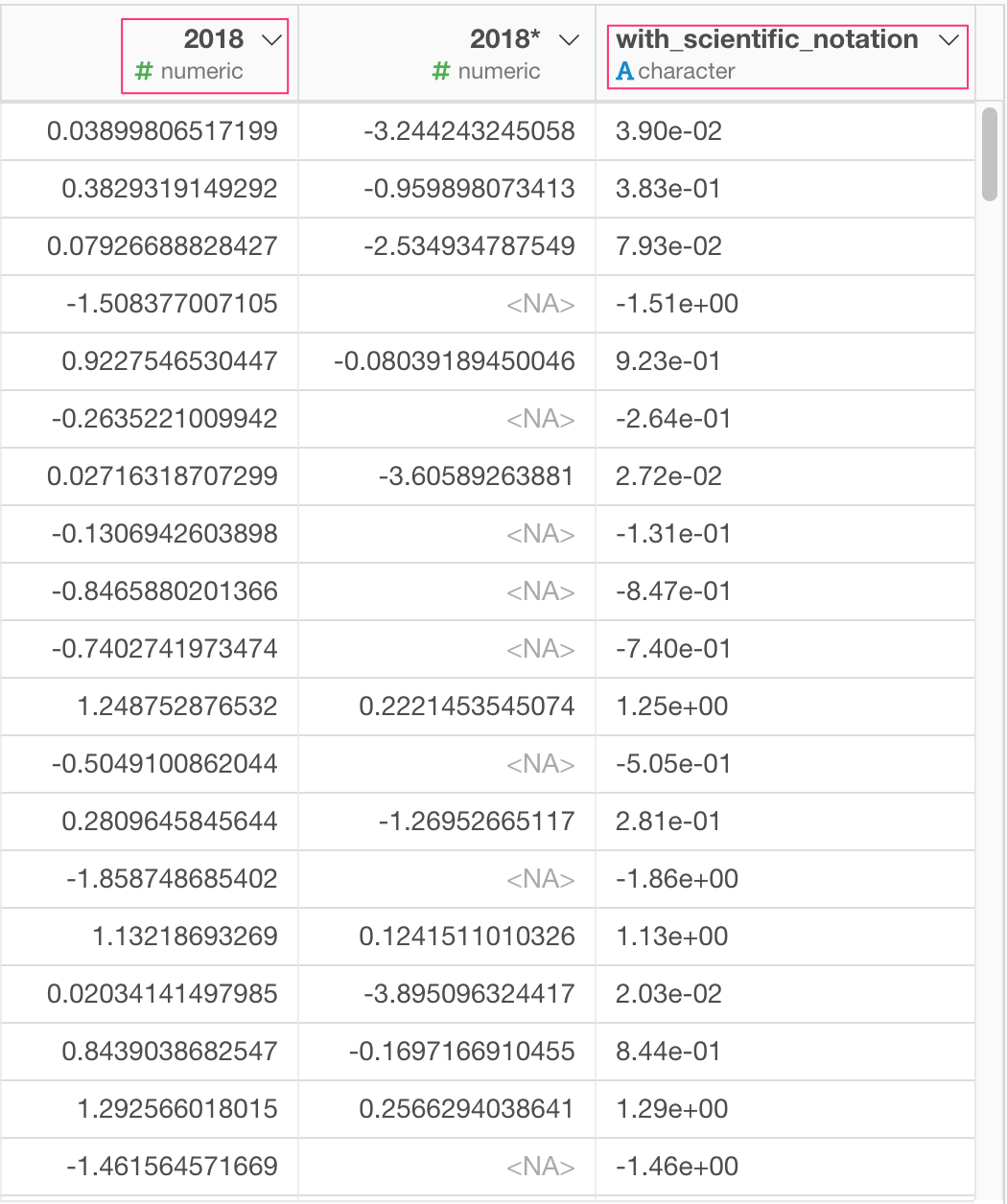# How to convert numeric value to scientific notation

If you have large numbers in your numeric column and want to convert those to scientific notation, you can do as follows.

## Create a new column with formatC

In R, there is a function called formatC which converts numeric value to text with specified format.

For example, if you have a column (2008) which contains numeric value, you can create a new text column like below.``formatC(`2008`, format = "e", digits = 2)``

format = “e” for the scientific notation and digit = 2 is for the desired number of digits after the decimal point.

This will create a new column like this